Tweeter Mids Fullrange Woofer no longer available PA Mids Woofer Fullrange no longer available Car Woofer no longer available

# Monacor

Monacor develops and produces products for HiFi, Car HiFi, PA, Multimedia and Home Cinema since 1965. The brands like MONACOR, IMG Stage Line and CARPOWER are well known for their high quality.

## Carpower Wooferrecommended cabinet 1:
closed cabinet with 6 L volume
from 98/62 Hz (-3dB/-8dB)

recommended cabinet 2:
23 L volume bass reflex cabinet
with HP70 reflex tube, 14 cm long.
from 49/39 Hz (-3dB/-8dB).

SP-167C       order no. mo-102790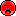- not available
16 cm bass driver with paper cone.
• power handling (continuous/programme) = 50/150 W
• frequency range = 58-5500 Hz
• resonance frequency fs = 49 Hz
• impedance R = 4 Ohm
• sound pressure level SPL = 91 dB (2,83V; 1m)
• DC resistance Re = 3,7 Ohm
• force factor BL = 5,6 N/A
• voice coil inductance L = 0,2 mH
• effective piston radiating area Sd = 137 cm2
• effective mechanical mass incl. air load mms = 12 g
• equivalent volume of compliance Vas = 22 l
• total Q factor Qts = 0,39 (Qms=2,89, Qes=0,45)
• voice coil diameter = 25 mm
• mounting diameter d = 148 mm
• overall diameter d = 170 mm
• mounting depth (not countersunk) t = 73,5 mmrecommended cabinet 1:
closed cabinet with 36 L volume
from 58/36 Hz (-3dB/-8dB)

recommended cabinet 2:
147 L volume bass reflex cabinet
with HP70 reflex tube, 3 cm long.
from 26/22 Hz (-3dB/-8dB).

SP-202C       order no. mo-102800- not available
19 cm bass driver with paper cone.
• power handling (continuous/programme) = 75/200 W
• frequency range = 48-4000 Hz
• resonance frequency fs = 37 Hz
• impedance R = 4 Ohm
• sound pressure level SPL = 91 dB (2,83V; 1m)
• DC resistance Re = 3,8 Ohm
• force factor BL = 5,51 N/A
• voice coil inductance L = 0,3 mH
• effective piston radiating area Sd = 214 cm2
• effective mechanical mass incl. air load mms = 19 g
• equivalent volume of compliance Vas = 61 l
• total Q factor Qts = 0,5 (Qms=3,64, Qes=0,58)
• voice coil diameter = 25 mm
• mounting diameter d = 182 mm
• overall diameter d = 202 mm
• mounting depth (not countersunk) t = 82 mmrecommended cabinet 1:
closed cabinet with 32 L volume
from 66/41 Hz (-3dB/-8dB)

recommended cabinet 2:
125 L volume bass reflex cabinet
with HP100 reflex tube, 9 cm long.
from 32/26 Hz (-3dB/-8dB).

SP-252C       order no. mo-102810- not available
24 cm bass driver with paper cone.
• power handling (continuous/programme) = 100/350 W
• frequency range = 40-3500 Hz
• resonance frequency fs = 35 Hz
• impedance R = 4 Ohm
• sound pressure level SPL = 93 dB (2,83V; 1m)
• DC resistance Re = 3,7 Ohm
• force factor BL = 8,08 N/A
• voice coil inductance L = 0,4 mH
• effective piston radiating area Sd = 353 cm2
• effective mechanical mass incl. air load mms = 38 g
• equivalent volume of compliance Vas = 96 l
• total Q factor Qts = 0,41 (Qms=3,15, Qes=0,47)
• voice coil diameter = 39 mm
• mounting diameter d = 231 mm
• overall diameter d = 256 mm
• mounting depth (not countersunk) t = 105,5 mm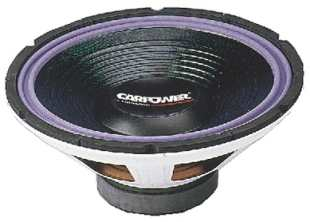SP-302C       order no. mo-102820- not available
29 cm bass driver with paper cone.
• power handling (continuous/programme) = 150/450 W
• frequency range = 35-3000 Hz
• resonance frequency fs = 33 Hz
• impedance R = 4 Ohm
• sound pressure level SPL = 93 dB (2,83V; 1m)
• DC resistance Re = 3,7 Ohm
• force factor BL = 7,99 N/A
• voice coil inductance L = 0,4 mH
• effective piston radiating area Sd = 531 cm2
• effective mechanical mass incl. air load mms = 62 g
• equivalent volume of compliance Vas = 155 l
• total Q factor Qts = 0,61 (Qms=4,19, Qes=0,71)
• voice coil diameter = 39 mm
• mounting diameter d = 282 mm
• overall diameter d = 305 mm
• mounting depth (not countersunk) t = 124 mm

## Carpower CRB Woofer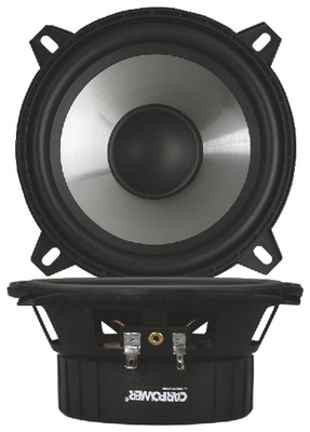CRB-130PS       order no. mo-131210       EUR 41,89excl. VAT: € 35.20 / \$ 36.26
13 cm bass-midrange driver with polypropylene cone.
• power handling (continuous/programme) = 30/60 W
• frequency range = 100-5000 Hz
• resonance frequency fs = 75 Hz
• impedance R = 4 Ohm
• sound pressure level SPL = 88 dB (2,83V; 1m)
• DC resistance Re = 3,6 Ohm
• force factor BL = 4 N/A
• voice coil inductance L = 0,6 mH
• effective piston radiating area Sd = 92 cm2
• effective mechanical mass incl. air load mms = 10 g
• equivalent volume of compliance Vas = 5,5 l
• total Q factor Qts = 0,98 (Qms=4,26, Qes=1,28)
• voice coil diameter = 25 mmCRB-165PS       order no. mo-131220       EUR 52,64excl. VAT: € 44.24 / \$ 45.56
16 cm bass-midrange driver with polypropylene cone.
• power handling (continuous/programme) = 35/70 W
• frequency range = 90-4500 Hz
• resonance frequency fs = 65 Hz
• impedance R = 4 Ohm
• sound pressure level SPL = 89 dB (2,83V; 1m)
• DC resistance Re = 3,6 Ohm
• force factor BL = 4,2 N/A
• voice coil inductance L = 0,6 mH
• effective piston radiating area Sd = 137 cm2
• effective mechanical mass incl. air load mms = 14,5 g
• equivalent volume of compliance Vas = 11 l
• total Q factor Qts = 0,99 (Qms=4,54, Qes=1,25)
• voice coil diameter = 25 mm

## Carpower Sonicopen a bigger photo

recommended cabinet 1:
closed cabinet with 11,8 L volume
from 51/32 Hz (-3dB/-8dB)

recommended cabinet 2:
48 L volume bass reflex cabinet
with HP70 reflex tube, 26 cm long.
from 24/20 Hz (-3dB/-8dB).

Sonic-10MK2, 2x2 Ohm       order no. mo-132190       - not available (_)
25 cm bass driver with aluminium cone. The basket is made of die-cast. The driver is made with a double voice coil and the given impedance values are for connection in series.
• power handling (continuous/programme) = 2x300/2x500 W
• frequency range = 45-300 Hz
• resonance frequency fs = 33 Hz
• impedance R = 4 Ohm
• sound pressure level SPL = 84 dB (2,83V; 1m)
• DC resistance Re = 2x1,9/1x3,8 Ohm
• force factor BL = 15,5 N/A
• voice coil inductance L = 2x0,6/1x2,4 mH
• effective piston radiating area Sd = 346 cm2
• effective mechanical mass incl. air load mms = 189 g
• equivalent volume of compliance Vas = 20 l
• total Q factor Qts = 0,49 (Qms=7,39, Qes=0,53)
• voice coil diameter = 75 mm
• mounting diameter d = 236 mm
• overall diameter d = 265 mm
• mounting depth (not countersunk) t = 194 mm
• mass m = 14,5 kgopen a bigger photo

Sonic-15MK2, 2x2 Ohm       order no. mo-132220       - not available (_)
0 cm bass driver with aluminium cone. The basket is made of die-cast. The driver is made with a double voice coil and the given impedance values are for connection in series.
• power handling (continuous/programme) = 2x400/2x800 W
• frequency range = 33-300 Hz
• resonance frequency fs = 22 Hz
• impedance R = 4 Ohm
• sound pressure level SPL = 87 dB (2,83V; 1m)
• DC resistance Re = 2x1,9/1x3,8 Ohm
• force factor BL = 18,88 N/A
• voice coil inductance L = 2x0,7/1x2,8 mH
• effective piston radiating area Sd = 830 cm2
• effective mechanical mass incl. air load mms = 400 g
• equivalent volume of compliance Vas = 119 l
• total Q factor Qts = 0,58 (Qms=6,82, Qes=0,63)
• voice coil diameter = 75 mm
• mass m = 15,7 kgopen a bigger photo

Sonic-12MK2, 2x2 Ohm       order no. mo-132200       - not available (_)
0 cm bass driver with aluminium cone. The basket is made of die-cast. The driver is made with a double voice coil and the given impedance values are for connection in series.
• power handling (continuous/programme) = 2x350/2x600 W
• frequency range = 35-300 Hz
• resonance frequency fs = 24 Hz
• impedance R = 4 Ohm
• sound pressure level SPL = 84 dB (2,83V; 1m)
• DC resistance Re = 2x1,9/1x3,8 Ohm
• force factor BL = 18 N/A
• voice coil inductance L = 2x0,7/1x2,8 mH
• effective piston radiating area Sd = 511 cm2
• effective mechanical mass incl. air load mms = 287 g
• equivalent volume of compliance Vas = 57 l
• total Q factor Qts = 0,49 (Qms=7,75, Qes=0,52)
• voice coil diameter = 75 mm
• mass m = 15,1 kg

## Carpower HQrecommended cabinet 1:
closed cabinet with 11,6 L volume
from 57/36 Hz (-3dB/-8dB)

recommended cabinet 2:
47 L volume bass reflex cabinet
with HP70 reflex tube, 22 cm long.
from 27/22 Hz (-3dB/-8dB).

CRB-25HQ       order no. mo-132090       - not available (_)
25 cm bass driver with high quality cone.
• power handling (continuous/programme) = 200/500 W
• frequency range = 50-300 Hz
• resonance frequency fs = 33 Hz
• impedance R = 4 Ohm
• sound pressure level SPL = 85 dB (2,83V; 1m)
• DC resistance Re = 3,3 Ohm
• force factor BL = 14,6 N/A
• voice coil inductance L = 2,2 mH
• effective piston radiating area Sd = 315 cm2
• effective mechanical mass incl. air load mms = 116 g
• equivalent volume of compliance Vas = 27 l
• total Q factor Qts = 0,45 (Qms=5,56, Qes=0,49)
• voice coil diameter = 38 mm
• mounting diameter d = 228 mm
• overall diameter d = 264 mm
• mounting depth (not countersunk) t = 144 mmrecommended cabinet 1:
closed cabinet with 20 L volume
from 57/36 Hz (-3dB/-8dB)

recommended cabinet 2:
77 L volume bass reflex cabinet
with HP100 reflex tube, 28 cm long.
from 27/22 Hz (-3dB/-8dB).

CRB-30HQ       order no. mo-132100       - not available (_)
30 cm bass driver with high quality cone.
• power handling (continuous/programme) = 250/600 W
• frequency range = 45-200 Hz
• resonance frequency fs = 31 Hz
• impedance R = 4 Ohm
• sound pressure level SPL = 88 dB (2,83V; 1m)
• DC resistance Re = 3,3 Ohm
• force factor BL = 14,6 N/A
• voice coil inductance L = 2,2 mH
• effective piston radiating area Sd = 492 cm2
• effective mechanical mass incl. air load mms = 157 g
• equivalent volume of compliance Vas = 55 l
• total Q factor Qts = 0,42 (Qms=3,66, Qes=0,48)
• voice coil diameter = 50 mm
• mounting diameter d = 278 mm
• overall diameter d = 315 mm
• mounting depth (not countersunk) t = 164 mmrecommended cabinet 1:
closed cabinet with 98 L volume
from 35/22 Hz (-3dB/-8dB)

recommended cabinet 2:
401 L volume bass reflex cabinet
with 2x HP100 reflex tube, 24 cm long.
from 16/14 Hz (-3dB/-8dB).

CRB-38HQ       order no. mo-132110       - not available (_)
37 cm bass driver with high quality cone.
• power handling (continuous/programme) = 300/700 W
• frequency range = 30-200 Hz
• resonance frequency fs = 22 Hz
• impedance R = 4 Ohm
• sound pressure level SPL = 87 dB (2,83V; 1m)
• DC resistance Re = 3,8 Ohm
• force factor BL = 15,6 N/A
• voice coil inductance L = 2,2 mH
• effective piston radiating area Sd = 779 cm2
• effective mechanical mass incl. air load mms = 257 g
• equivalent volume of compliance Vas = 177 l
• total Q factor Qts = 0,49 (Qms=3,95, Qes=0,56)
• voice coil diameter = 60 mm
• mounting diameter d = 348 mm
• overall diameter d = 390 mm
• mounting depth (not countersunk) t = 189 mm

## Carpower Predatorrecommended cabinet 1:
closed cabinet with 3,5 L volume

recommended cabinet 2:
13,8 L volume bass reflex cabinet
with HP70 reflex tube, 14 cm long.
from 60/49 Hz (-3dB/-8dB).

PREDATOR-6/4       order no. mo-131470       - not available (_)
16 cm bass driver with carbon cone. The basket is made of die-cast. To avoid compression effects the driver possesses a pole piece hole.
• power handling (continuous/programme) = 100/180 W
• frequency range = 90-2500 Hz
• resonance frequency fs = 66 Hz
• impedance R = 4 Ohm
• sound pressure level SPL = 91 dB (2,83V; 1m)
• DC resistance Re = 3,15 Ohm
• force factor BL = 6 N/A
• voice coil inductance L = 0,4 mH
• effective piston radiating area Sd = 127 cm2
• effective mechanical mass incl. air load mms = 12,4 g
• equivalent volume of compliance Vas = 10,6 l
• total Q factor Qts = 0,41 (Qms=4,97, Qes=0,45)
• voice coil diameter = 25 mm
• mounting diameter d = 144 mm
• overall diameter d = 180 mm
• mounting depth (not countersunk) t = 78 mm

## Carpower Pulsarrecommended cabinet 1:
closed cabinet with 11,4 L volume
from 57/36 Hz (-3dB/-8dB)

recommended cabinet 2:
47 L volume bass reflex cabinet
with HP70 reflex tube, 22 cm long.
from 26/22 Hz (-3dB/-8dB).

PULSAR-10       order no. mo-132060       - not available (_)
25 cm bass driver with high quality cone. The basket is made of die-cast. The voice coil is made of kapton.
• power handling (continuous/programme) = 2x175/2x250 W
• frequency range = 50-300 Hz
• resonance frequency fs = 35 Hz
• impedance R = 4 Ohm
• sound pressure level SPL = 86 dB (2,83V; 1m)
• DC resistance Re = 2x1,9/1x3,8 Ohm
• force factor BL = 15,7 N/A
• voice coil inductance L = 2x0,6mh/1,24mh mH
• effective piston radiating area Sd = 360 cm2
• effective mechanical mass incl. air load mms = 148 g
• equivalent volume of compliance Vas = 22 l
• total Q factor Qts = 0,48 (Qms=5,92, Qes=0,52)
• voice coil diameter = 50 mm
• mounting diameter d = 236 mm
• overall diameter d = 262 mm
• mounting depth (not countersunk) t = 75 mmPULSAR-12       order no. mo-132070       - not available (_)
30 cm bass driver with high quality cone. The basket is made of die-cast. The voice coil is made of kapton.
• power handling (continuous/programme) = 2x200/2x350 W
• frequency range = 50-300 Hz
• resonance frequency fs = 35 Hz
• impedance R = 4 Ohm
• sound pressure level SPL = 86 dB (2,83V; 1m)
• DC resistance Re = 2x1,9/1x3,8 Ohm
• force factor BL = 15,7 N/A
• effective piston radiating area Sd = 510 cm2
• effective mechanical mass incl. air load mms = 275 g
• equivalent volume of compliance Vas = 25 l
• total Q factor Qts = 0,64 (Qms=6,11, Qes=0,71)
• voice coil diameter = 75 mm
• mounting diameter d = 267 mm
• overall diameter d = 318 mm
• mounting depth (not countersunk) t = 90 mm

## Carpower Raptor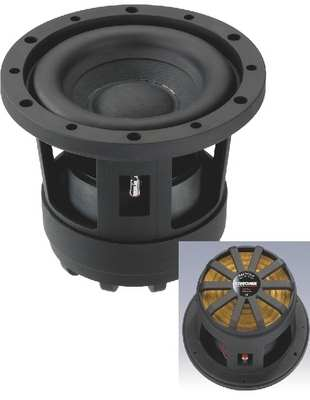open a bigger photo

RAPTOR-6       order no. mo-104350       EUR 185,94excl. VAT: € 156.25 / \$ 160.94
16 cm bass driver with high quality cone.
• power handling (continuous/programme) = 100/300 W
• frequency range = 70-800 Hz
• resonance frequency fs = 53 Hz
• impedance R = 4 Ohm
• sound pressure level SPL = 88 dB (2,83V; 1m)
• DC resistance Re = 3,4 Ohm
• force factor BL = 7,6 N/A
• voice coil inductance L = 0,6 mH
• effective piston radiating area Sd = 113 cm2
• effective mechanical mass incl. air load mms = 23 g
• equivalent volume of compliance Vas = 6,9 l
• total Q factor Qts = 0,36 (Qms=2,2, Qes=0,43)
• voice coil diameter = 25 mm
• mounting diameter d = 144 mm
• overall diameter d = 182 mm
• mounting depth (not countersunk) t = 135,5 mmrecommended cabinet 1:
closed cabinet with 23 L volume
from 50/32 Hz (-3dB/-8dB)

recommended cabinet 2:
93 L volume bass reflex cabinet
with HP100 reflex tube, 30 cm long.
from 23/19 Hz (-3dB/-8dB).

RAPTOR-12MK2       order no. mo-100210       - not available (_)
30 cm bass driver with aluminium cone.
• power handling (continuous/programme) = 1000/1500 W
• frequency range = 50-300 Hz
• resonance frequency fs = 34 Hz
• impedance R = 4 Ohm
• sound pressure level SPL = 87 dB (2,83V; 1m)
• DC resistance Re = 3,4 Ohm
• force factor BL = 16,6 N/A
• voice coil inductance L = 1,1 mH
• effective piston radiating area Sd = 470 cm2
• effective mechanical mass incl. air load mms = 211 g
• equivalent volume of compliance Vas = 32 l
• total Q factor Qts = 0,52 (Qms=7,87, Qes=0,56)
• voice coil diameter = 77 mm
• mounting diameter d = 282 mm
• overall diameter d = 320 mm
• mounting depth (not countersunk) t = 227 mm
• mass m = 13,1 kg
PDF data sheetRAPTOR-15MK2       order no. mo-100220       - not available (_)
38 cm bass driver with aluminium cone.
• power handling (continuous/programme) = 1000/1500 W
• frequency range = 45-300 Hz
• resonance frequency fs = 31 Hz
• impedance R = 4 Ohm
• sound pressure level SPL = 89 dB (2,83V; 1m)
• DC resistance Re = 3,4 Ohm
• force factor BL = 15,53 N/A
• voice coil inductance L = 1,1 mH
• effective piston radiating area Sd = 810 cm2
• effective mechanical mass incl. air load mms = 260 g
• equivalent volume of compliance Vas = 87 l
• total Q factor Qts = 0,69 (Qms=8,5, Qes=0,75)
• voice coil diameter = 77 mm
• mounting diameter d = 361 mm
• overall diameter d = 397 mm
• mounting depth (not countersunk) t = 267 mm
• mass m = 13,8 kg
PDF data sheet

## Shaker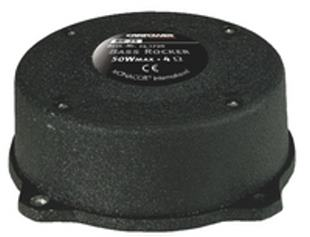BR-25       order no. mo-141790       - not available (_)
Bass Shaker.
• power handling (continuous/programme) = 50 W
• frequency range = 50-300 Hz
• resonance frequency fs = 40 Hz
• impedance R = 4 Ohm
• mass m = 1,5 kg
PDF data sheet
 Tweeter Mids Fullrange Woofer no longer available PA Mids Woofer Fullrange no longer available Car Woofer no longer available
The cabinet volume calculations are executed considering an external resistance of 0.3 Ohm. We are using, if no other data is known or available, the information supplied by the manufacturer.

Please note: the frequency response doesn't say much about the cut-off frequency inside the cabinet! Therefore, we mostly give you 2 examples with dimensioning considerations and an indication, how low the loudspeaker will reproduce.

Home